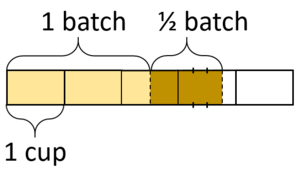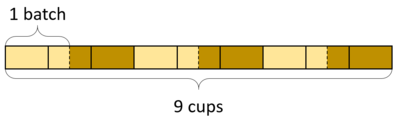# How Many Batches/What Fraction of a Batch?

Alignments to Content Standards: 6.NS.A

A batch of cookies requires 2 cups of flour. How many cups are needed for 3 batches? Here are a diagram and a multiplication equation that represent this situation:$$3 \times 2 = ?$$

Draw a diagram and write a multiplication equation for each situation.

1. A batch of cupcakes requires $1 \frac12$ cups of flour. How many cups are needed for 3 batches?
2. A batch of bread requires 3 cups of flour. How many cups are needed for $\frac12$ of a batch?
3. A batch of rolls requires $2\frac12$ cups of flour. How many cups are needed for $1\frac12$ batches?

A batch of cookies requires 2 cups of flour. How many batches can you make with 8 cups of flour? Here are a diagram and a division equation that represent this situation:$$8 \div 2 = ?$$

Draw a diagram and write a division equation for each situation.

1. A batch of cupcakes requires $1 \frac12$ cups of flour. How many batches can you make with 9 cups of flour?
2. A batch of bread requires 3 cups of flour. How many batches can you make with 2 cups of flour?
3. A batch of rolls requires $2\frac12$ cups of flour. How many batches can you make with $4\frac12$ cups of flour?

## IM Commentary

The purpose of this task is to help students extend their understanding of multiplication and division of whole numbers to multiplication and division of fractions. In grade 5, students

• multiply two fractions together and learn that a fraction times a number can be interpreted as that fraction of the number,
• divide two whole numbers with a non-whole number quotient, and
• divide a whole number by a unit fraction and a unit fraction by a whole number.

Notably, all of the division students do in grade 5 can be supported by an understanding of a whole number of groups. The big shift in grade 6 is that students tackle division contexts that involve a fractional number of groups.

The task does not ask students to find the product or quotient since the task is more about learning how to represent the situation, but teachers might choose to ask students to find or estimate the answers, if desired.

This task helps students see how to represent How Many Groups/What Fraction of a Group? contexts with division equations. It's designed to be used along with the task How Much in One Batch? which covers the How Much in a Group? situation.

To offload some of the tedium of drawing diagrams so that students can focus on thinking about sizes and numbers of batches, teachers might offer some printed templates for students to work on.

## Solution

(Note: In all the diagrammed solutions, a solid line shows a division in cups and a dashed line shows a division in batches.)

1. A batch of cupcakes requires $1 \frac12$ cups of flour. How many cups needed for 3 batches?$$3 \times 1\frac12 = ?$$
2. A batch of bread requires 3 cups of flour. How many cups needed for $\frac12$ a batch?$$\frac12 \times 3 = ?$$
3. A batch of rolls requires $2\frac12$ cups of flour. How many cups needed for $1\frac12$ batches? (In this diagram, the dotted lines are used to show the partition of one of the cups into four parts.)$$1\frac12 \times 2\frac12 = ?$$

1. A batch of cupcakes requires $1 \frac12$ cups of flour. How many batches can you make with 9 cups of flour?$$9 \div 1\frac12 = ?$$
2. A batch of bread requires 3 cups of flour. How many batches can you make with 2 cups of flour?$$2\div 3 = ?$$
3. A batch of rolls requires $2\frac12$ cups of flour. How many batches can you make with $4\frac12$ cups of flour?$$4 \frac12 \div 2 \frac12 = ?$$